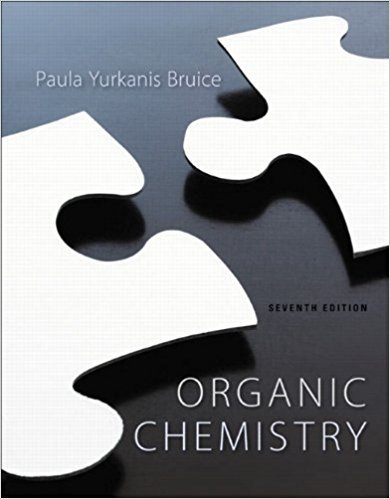> > > Chapter 13

# Solutions for Chapter 13: Organic Chemistry 7th Edition## Full solutions for Organic Chemistry | 7th Edition

ISBN: 9781269406772Solutions for Chapter 13

Solutions for Chapter 13
4 5 0 315 Reviews
25
0
##### ISBN: 9781269406772

Chapter 13 includes 68 full step-by-step solutions. This expansive textbook survival guide covers the following chapters and their solutions. Organic Chemistry was written by and is associated to the ISBN: 9781269406772. Since 68 problems in chapter 13 have been answered, more than 108966 students have viewed full step-by-step solutions from this chapter. This textbook survival guide was created for the textbook: Organic Chemistry, edition: 7.

Key Chemistry Terms and definitions covered in this textbook
• Achiral

An object that lacks chirality; an object that has no handedness

• Aldehyde

A compound containing a !CHO group

• back-side attack

In SN2 reactions, the side opposite the leaving group, which is where the nucleophile attacks.

• calorie

A unit of energy; it is the amount of energy needed to raise the temperature of 1 g of water by 1 °C from 14.5 °C to 15.5 °C. A related unit is the joule: 1 cal = 4.184 J. (Section 5.1)

• cell voltage.

Difference in electrical potential between the anode and the cathode of a galvanic cell. (18.2)

• chemical kinetics

The area of chemistry concerned with the speeds, or rates, at which chemical reactions occur. (Chapter 14: Introduction)

• denatured protein.

Protein that does not exhibit normal biological activities. (25.3)

• diastereotopic

Nonequivalent protons for which the replacement test produces diastereomers.

• electron shell

A collection of orbitals that have the same value of n. For example, the orbitals with n = 3 (the 3s, 3p, and 3d orbitals) comprise the third shell. (Section 6.5)

• electronic structure

The arrangement of electrons in an atom or molecule. (Chapter 6:Introduction)

• Energy diagram

A graph showing the changes in energy that occur during a chemical reaction; energy is plotted on the vertical axis, and reaction progress is plotted on the horizontal axis. Also called a reaction coordinate diagram

• excited state

A state that is achieved when a compound absorbs energy.

• Haworth projection

For substituted cycloalkanes, a drawing style used to clearly identify which groups are above the ring and which groups are below the ring. (See also Sect. 4.14.)

• nucleon

A particle found in the nucleus of an atom. (Section 21.1)

Addition of a reagent to a metal center causing it to add two substituents and to increase its oxidation state by two

• photoelectric effect

The emission of electrons from a metal surface induced by light. (Section 6.2)

• Plane-polarized light

Light oscillating in only parallel planes.

• proton

A positively charged subatomic particle found in the nucleus of an atom. (Section 2.3)

• reactant

A starting substance in a chemical reaction; it appears to the left of the arrow in a chemical equation. (Section 3.1)

• Sigma (s) molecular orbital

A molecular orbital in which electron density is concentrated between two nuclei, along the axis joining them, and is cylindrically symmetrical

×

I don't want to reset my password

Need help? Contact support

Need an Account? Is not associated with an account
We're here to help